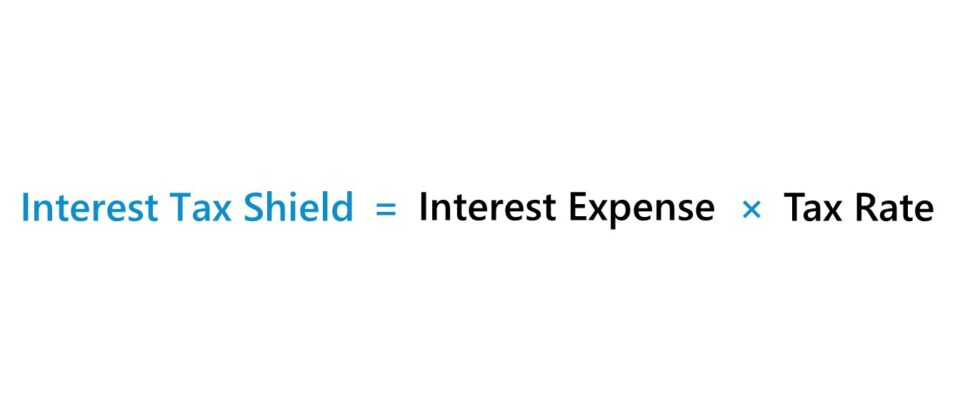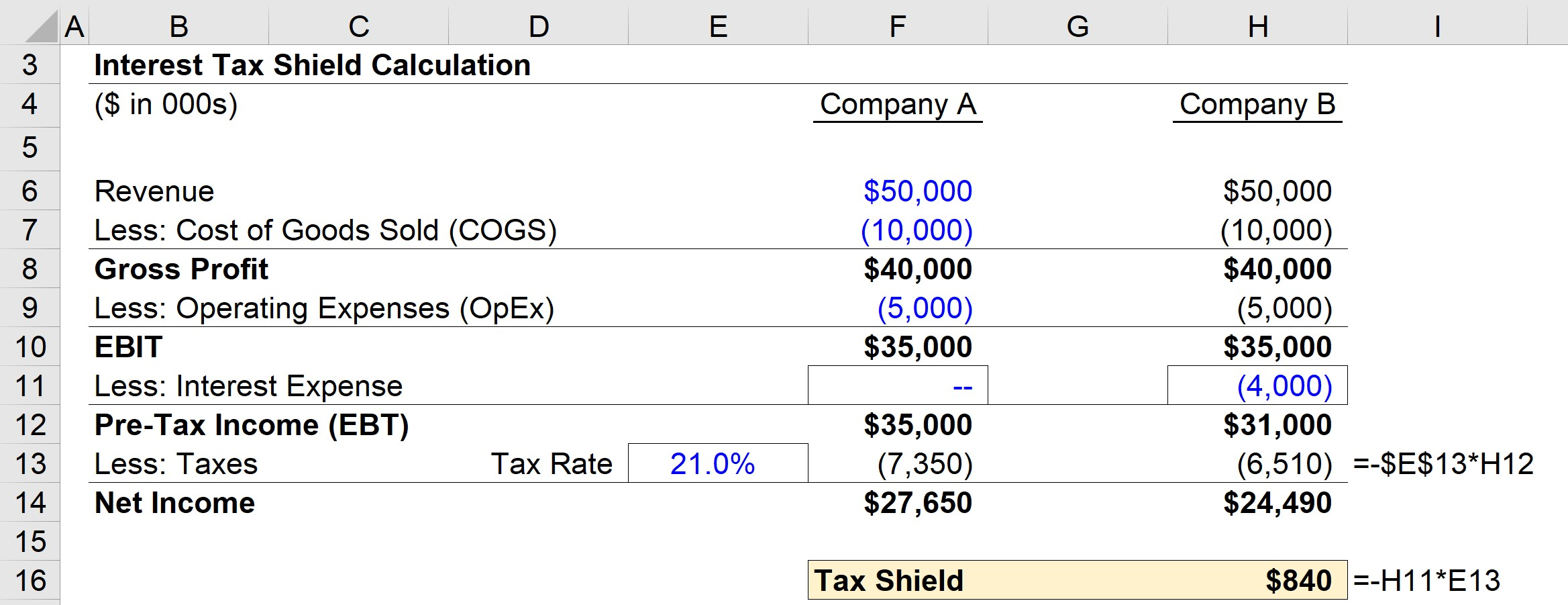# Interest Tax Shield

Guide to Understanding the Interest Tax Shield## How to Calculate Interest Tax Shield (Step-by-Step)

If a company decides to take on debt, the lender is compensated through interest expense, which will be reflected on the company’s income statement in the non-operating income/(expenses) section.

The interest tax shield helps offset the loss caused by the interest expense associated with debt, which is why companies pay close attention to it when taking on more debt.

Because of the tax-deductibility of interest expense, the weighted average cost of capital (WACC) takes into account the tax reduction in its formula. Unlike dividends, interest expense payments reduce the taxable income.

Neglecting the tax shield would be ignoring a very important benefit of borrowing that could potentially lead to a company being undervalued from the inflated cost of debt.

But since the WACC already factors this in, the calculation of unlevered free cash flow does NOT account for these tax savings – otherwise, you’d be double-counting the benefit.

For this reason, the formula for measuring a company’s unlevered free cash flow starts with the net operating profit after taxes (NOPAT), which taxes the operating income metric, as opposed to using a levered metric (i.e. post-interest).

The value of a tax shield can be calculated as the total amount of the taxable interest expense multiplied by the tax rate.

## Tax Shield Formula

The formula for calculating the interest tax shield is as follows.

Interest Tax Shield = Interest Expense × Tax Rate

For instance, if the tax rate is 21.0% and the company has \$1m of interest expense, the tax shield value of the interest expense is \$210k (21.0% x \$1m).

Note that the following formula above is only applicable for companies that are already profitable at the taxable income line.

Since the interest expense on debt is tax-deductible, whereas dividends to common equity holders are not, debt financing is often considered to be a “cheaper” source of capital initially.

Therefore, companies seek to maximize the tax benefits of debt without being at risk of default (i.e. failing to meet interest expense or principal repayment obligations on the date due).

## Interest Tax Shield Calculator – Excel Template

We’ll now move to a modeling exercise, which you can access by filling out the form below.Submitting ...

## Step 1. Operating Assumptions

In this exercise, we’ll be comparing the net income of a company with vs without interest expense payments. For both companies, we’ll be using the following operating assumptions:

Here, Company A will carry no debt on its balance sheet (and thus have zero interest expense), whereas Company B will have \$4m in interest expense.

For both companies, the financials are the same until the operating income (EBIT) line, where each has an EBIT of \$35m.

## Step 2. Interest Tax Shield Calculation Analysis

But once the interest expense is accounted for, the two companies’ financials begin to differ. Since Company A has no non-operating expenses to factor in, its taxable income remains at \$35m.

On the other hand, Company B’s taxable income becomes \$31m after deducting the \$4m in interest expense.

Given the decreased taxable income, Company B’s taxes for the current period are approximately \$6.5m, which is \$840k lower than Company A’s \$7.4m in taxes.

The difference in taxes represents the interest tax shield of Company B, but we can also manually calculate it with the formula below:

• Interest Tax Shield = Interest Expense Deduction x Effective Tax Rate
• Interest Tax Shield = \$4m x 21% = \$840k

While Company A does have a higher net income, all else being equal, Company B would have more cash on hand from its debt financing that can be spent on future growth plans, benefiting from the tax savings on interest expense.In conclusion, we can see the effects of the interest tax shield from our simple comparison of two companies with two different capital structures.

As shown in the completed output above, Company B’s taxes were \$840k lower than Company A’s taxes.Step-by-Step Online Course

### Everything You Need To Master Financial Modeling

Enroll in The Premium Package: Learn Financial Statement Modeling, DCF, M&A, LBO and Comps. The same training program used at top investment banks.Inline FeedbacksLearn Financial Modeling Online

Everything you need to master financial and valuation modeling: 3-Statement Modeling, DCF, Comps, M&A and LBO.

X

The Wall Street Prep Quicklesson Series

7 Free Financial Modeling Lessons

Get instant access to video lessons taught by experienced investment bankers. Learn financial statement modeling, DCF, M&A, LBO, Comps and Excel shortcuts.